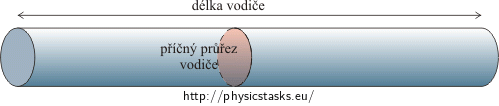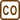## Electrical Resistances of Conductors of Different Lengths

Two wires made from the same material weigh the same. The first conductor is n-times longer than the other. What is the ratio between the electrical resistances of the two wires?

• #### Hint 1

Remember or look up how to calculate the resistance of a conductor based on its shape (dimensions).

• #### Hint 2

The two wires are made of the same material, have the same weight and the first wire is longer than the second one. In what else must the two wires differ?

What is the volume of the two wires made of the same amount of the same material?

• #### Analysis

The electrical resistance of a conductor is inversely proportional to its cross-sectional area and directly proportional to its length and to the resistivity, which characterizes the material of the conductor.

The resistivity of two conductors made of the same material is the same. The ratio of their resistances thus depends on their lengths and cross-sectional areas. The ratio of the resistances of the two conductors is directly proportional to the ratio of their lengths and inversely proportional to the ratio of their cross-sectional areas.

$\frac{R_1}{R_2} \propto \frac{l_1}{l_2}$ $\frac{R_1}{R_2} \propto \frac{A_2}{A_1}$

The wires in the assignment of the task are not only made from the same material but also have the same weight. It follows that the volumes of the two conductors are also equal. A wire can be thought of as a long cylinder of a given length and certain cross-sectional area. The volume of such wire is equal to the product of its length and cross-sectional area.By equaling the volumes of the two conductors, we find that the ratio of their lengths is equal to the inverse ratio of their cross-sectional areas. (This means that the n-times longer conductor has n-times smaller cross-sectional area than the other conductor.)

Now, we relate this consideration to the relation we have found by comparing the resistances of the two conductors. Because the first conductor is n-times longer and the second conductor has n-times larger cross-sectional area, we find that the ratio of the resistances of the two conductors is equal to n2.

• #### Solution

Two wires made of the same material (i.e. having the same density) and of the same weight must have the same volume V

We calculate the volume as the product of length l and cross-sectional area A:

$V\,=\,l\cdot A\,.$

Since the volumes of the two wires are equal:

$V_1\,=\,V_2,$

it follows that:

$l_1\,A_1\,=\,l_2\,A_2$ $\frac{l_1}{l_2}\,=\,\frac{A_2}{A_1}\,. \tag{1}$

The assignment states that the first wire is n-times longer than the second one:

$l_1\,=\,n\cdot l_2\,. \tag{2}$

The lengths thus are in a ratio:

$\frac{l_1}{l_2}\,=\,\frac{n\cdot l_2}{l_2}\,=\,n\,.$

We substitute this ratio into equation (1) and we see that the cross-sectional arae of the second wire must be n-times larger than that of the first wire:

$\frac{l_1}{l_2}\,=\,\frac{A_2}{A_1}\,=\,n$ $A_2\,=\,n\cdot A_1\,. \tag{3}$

Let us now express the electrical resistance R of a wire in dependence to its properties:

$R\,=\,\rho\,\frac{l}{A}\,\mathrm{,}$

where ρ is the resistivity (or specific eletrical resistance) of the conductor, l is its length and A is its cross-sectional area.

Both wires are made of the same material — they have the same resistivities. By comparing the resistances of the two wires we get the following formula:

$\frac{R_1}{R_2}\,=\,\frac{\rho\frac{l_1}{A_1}}{\rho\frac{l_2}{A_2}}\,=\,\frac{\frac{l_1}{A_1}}{\frac{l_2}{A_2}}\,,$

or:

$\frac{R_1}{R_2}\,=\,\frac{l_1 A_2}{l_2 A_1}\,.$

We substitute ratios (2) and (3) into the last formula:

$\frac{R_1}{R_2}\,=\,\frac{l_1A_2}{l_2A_1}\,=\,\frac{n\cdot l_2 \cdot n\cdot A_1}{l_2 \cdot A_1}\,=\,n^2\,.$

And we see that the resistance of the first wire is n2-times higher than the resistance of the second wire:

$R_1\,=\,n^2\cdot R_2\,.$